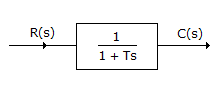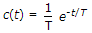# Electronics and Communication Engineering - Automatic Control Systems

### Exercise :: Automatic Control Systems - Section 6

36.

Assertion (A): Derivative control action is also called rate control and has an anticipatory character.

Reason (R): Derivative control amplifies noise signals.

 A. Both A and R are correct and R is correct explanation of A B. Both A and R are correct but R is not correct explanation of A C. A is correct but R is wrong D. R is correct but A is wrong

Explanation:

No answer description available for this question. Let us discuss.

37.

Assertion (A): When a PID controller is added to a system, the peak overshoot to step input cannot be determined easily.

Reason (R): Use of PID controller introduces a zero in the forward path.

 A. Both A and R are correct and R is correct explanation of A B. Both A and R are correct but R is not correct explanation of A C. A is correct but R is wrong D. R is correct but A is wrong

Explanation:

No answer description available for this question. Let us discuss.

38.

For the system shown in the given figure the time response to unit step input isA.B. c(t) = T(1 - e-t/T) C. c(t) = 1 - e-t/T D. c(t) = T e-t/T

Explanation:

No answer description available for this question. Let us discuss.

39.

The slope of log magnitude asymptote changes by - 40 dB/ decade at a certain frequency and the error between asymptote and actual curve is - 6 dB. This means that

 A. a double pole is present B. a pair of complex conjugate poles is present C. either a double pole or a pair of complex conjugate poles is present D. either a pole or zero at that frequency is present

Explanation:

No answer description available for this question. Let us discuss.

40.

Friction forces acts in a direction

 A. opposite to that of velocity B. same as that of velocity C. at right angle to that of velocity D. partly opposite and partly at right angle to the direction of velocity